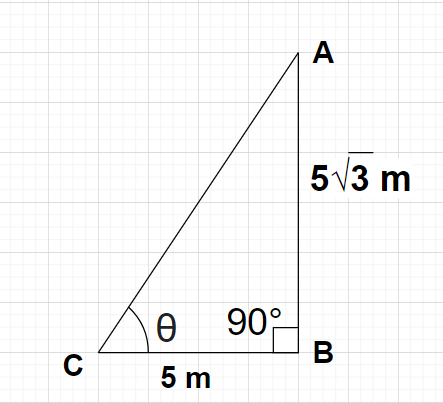Courses
Courses for Kids
Free study material
Offline Centres
MoreLast updated date: 27th Nov 2023
Total views: 379.2k
Views today: 11.79k

# A tower is $5\sqrt 3$ meter high. Find the angle of elevation of its top from a point 5 meter away from its foot.Verified
379.2k+ views
Hint- Here, we will be making diagram according to the problem statement and then we will use the formula for tangent trigonometric function i.e, $\tan \theta = \dfrac{{{\text{Perpendicular}}}}{{{\text{Base}}}}$ in order to evaluate the value for the angle of elevation (i.e., $\theta$).

Given, height of the tower AB = $5\sqrt 3$ meter
Let us suppose that the angle of elevation of the top of the tower (i.e., point A) from point C is $\theta$.
As we know that in any right angled triangle, $\tan \theta = \dfrac{{{\text{Perpendicular}}}}{{{\text{Base}}}}{\text{ }} \to {\text{(1)}}$
$\tan \theta = \dfrac{{{\text{AB}}}}{{{\text{BC}}}} = \dfrac{{5\sqrt 3 }}{5} \\ \Rightarrow \tan \theta = \sqrt 3 {\text{ }} \to {\text{(2)}} \\$
Also we know that $\tan {60^0} = \sqrt 3 {\text{ }} \to {\text{(3)}}$
i.e., $\tan \theta = \tan {60^0} \\ \Rightarrow \theta = {60^0} \\$
Therefore, the required angle of elevation of the top of the tower from a point 5 meter away from the foot of the tower is ${60^0}$.
Note- In any right angled triangle, the hypotenuse is the side opposite to ${90^0}$ (in this case the right angle is at B and the side opposite to vertex B is AC), the perpendicular is the side opposite to the considered angle $\theta$ (in this case the perpendicular is AB) and the base is the remaining side (in this case base is BC).